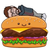# New to Qlik Sense

If you’re new to Qlik Sense, start with this Discussion Board and get up-to-speed quickly.

Announcements
Support Case Portal has moved to Qlik Community! Read the FAQs to start exploring Support resources.
cancel
Showing results for
Did you mean:Creator II

## count of percentage

Team in Qlik Sense.  How can I get the count() of records where the percentage of budget is over 75%.

count(num(TRIM(sum(BH_AMOUNT_ACT + BH_AMOUNT_GLA + BH_AMOUNT_GLL + BH_AMOUNT_GLQ + )) / sum(BH_AMOUNT_BUD) *100) > 75)

Labels (2)

• ### Percentages

1 Solution

Accepted SolutionsCreator III

Are all the BH_* fields in the same table?

I would flag the Projects/Records that are under/over budget in the load script, and then you can use set analysis to do the visualization?

Or attach the % in the load script and then you can use set analysis to count > 0.75

If you are looking to use in a KPI, does :

If ( (sum(BH_AMOUNT_ACT + BH_AMOUNT_GLA + BH_AMOUNT_GLL + BH_AMOUNT_GLQ + BH_AMOUNT_GLR + BH_AMOUNT_GLE + BH_AMOUNT_PAY + BH_AMOUNT_OTHER) * 100 / sum(BH_AMOUNT_BUD)) => 75, count(record_id) )

work?

You could also flag each entry in the table with the same formula and then the table total would be the Count.

Eg.

If ( (sum(BH_AMOUNT_ACT + BH_AMOUNT_GLA + BH_AMOUNT_GLL + BH_AMOUNT_GLQ + BH_AMOUNT_GLR + BH_AMOUNT_GLE + BH_AMOUNT_PAY + BH_AMOUNT_OTHER) * 100 / sum(BH_AMOUNT_BUD)) => 75, 1, 0 )

5 RepliesCreator III

I see some syntax errors with your formula.

Why is there a trailing + in the sum? and why TRIM? Why num?

You ask for a count but you don't have a field specified to count from...

Are those fields not loaded as numbers?

I would try

If(

((BH_AMOUNT_ACT + BH_AMOUNT_GLA + BH_AMOUNT_GLL + BH_AMOUNT_GLQ)  / (BH_AMOUNT_BUD)) > 0.75,

Count(records_unique_field)

)

The better way would be to use the data script and load the % of budget as a field.Creator II
Author

Sorry i am new at this.

if(sum(BH_AMOUNT_ACT + BH_AMOUNT_GLA + BH_AMOUNT_GLL + BH_AMOUNT_GLQ + BH_AMOUNT_GLR + BH_AMOUNT_GLE + BH_AMOUNT_PAY + BH_AMOUNT_OTHER) > 0
,if(sum(BH_AMOUNT_BUD) > 0, sum(BH_AMOUNT_ACT + BH_AMOUNT_GLA + BH_AMOUNT_GLL + BH_AMOUNT_GLQ + BH_AMOUNT_GLR + BH_AMOUNT_GLE + BH_AMOUNT_PAY + BH_AMOUNT_OTHER)
/ sum(BH_AMOUNT_BUD)*100
))

The above I am using in the row column of my grid. I'm not happy with the format (number, format '0.00%') BUT, the bigger issue is they want a total of how many records are over 75% of the budget or more.

count(distinct PROJECT_ID)

2 questions:  Best way to format/show percentage.  & How to get percentage of records that are >=75%Creator II
Author

Much closer:

sum(BH_AMOUNT_ACT + BH_AMOUNT_GLA + BH_AMOUNT_GLL + BH_AMOUNT_GLQ + BH_AMOUNT_GLR + BH_AMOUNT_GLE + BH_AMOUNT_PAY + BH_AMOUNT_OTHER) * 100 / sum(BH_AMOUNT_BUD)Creator III

Are all the BH_* fields in the same table?

I would flag the Projects/Records that are under/over budget in the load script, and then you can use set analysis to do the visualization?

Or attach the % in the load script and then you can use set analysis to count > 0.75

If you are looking to use in a KPI, does :

If ( (sum(BH_AMOUNT_ACT + BH_AMOUNT_GLA + BH_AMOUNT_GLL + BH_AMOUNT_GLQ + BH_AMOUNT_GLR + BH_AMOUNT_GLE + BH_AMOUNT_PAY + BH_AMOUNT_OTHER) * 100 / sum(BH_AMOUNT_BUD)) => 75, count(record_id) )

work?

You could also flag each entry in the table with the same formula and then the table total would be the Count.

Eg.

If ( (sum(BH_AMOUNT_ACT + BH_AMOUNT_GLA + BH_AMOUNT_GLL + BH_AMOUNT_GLQ + BH_AMOUNT_GLR + BH_AMOUNT_GLE + BH_AMOUNT_PAY + BH_AMOUNT_OTHER) * 100 / sum(BH_AMOUNT_BUD)) => 75, 1, 0 )Creator II
Author

If((sum(BH_AMOUNT_ACT + BH_AMOUNT_GLA + BH_AMOUNT_GLL + BH_AMOUNT_GLQ + BH_AMOUNT_GLR + BH_AMOUNT_GLE + BH_AMOUNT_PAY + BH_AMOUNT_OTHER) * 100 / sum(BH_AMOUNT_BUD)) > 0.75,
Count(distinct PROJECT_ID))Tags
Community Browser